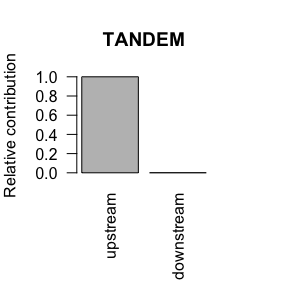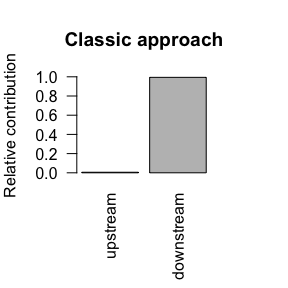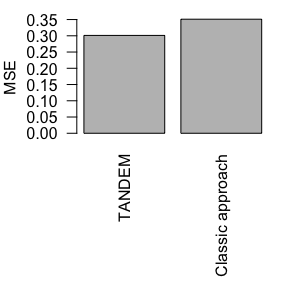# TANDEM

#### 2017-06-14

TANDEM is a two-stage regression method that can be used when various input data types are correlated, for example gene expression and methylation in drug response prediction. In the first stage it uses the upstream features (such as methylation) to predict the response variable (such as drug response), and in the second stage it uses the downstream features (such as gene expression) to predict the residuals of the first stage. In our manuscript (Aben et al., 2016), we show that using TANDEM prevents the model from being dominated by gene expression and that the features selected by TANDEM are more interpretable.

This vignette provides a code example for various parts that covers all parts of the package. The example starts by loading an artificial data example.

library(TANDEM)
library(glmnet)
x = example_data$x y = example_data$y
upstream = example_data$upstream data_types = example_data$data_types

To fit a TANDEM model and query the result (coefficients and predictions), you can use the following code. We tried to make the interface as comparable to glmnet as possible.

# fit a tandem model, determine the coefficients and create a prediction
fit = tandem(x, y, upstream, alpha=0.5)
beta = coef(fit)
y_hat = predict(fit, newx=x)

In the next part we’ll be comparing TANDEM with glmnet. To make this comparison fair, we fix the cross-validation folds, so that we can use the same folds for both methods.

# fix the cv folds, to facilitate a comparison between models
set.seed(1)
n = nrow(x)
nfolds = 10
foldid = ceiling(sample(1:n)/n * nfolds)

fit = tandem(x, y, upstream, alpha=0.5, foldid=foldid)
fit2 = cv.glmnet(x, y, alpha=0.5, foldid=foldid)

We’ll compare the two methods in two ways. First, we’ll look at the relative contribution of the data types. Note that TANDEM is able to predict the response from upstream data, while the classic approach (glmnet), requires downstream features.

# assess the relative contribution of upstream and downstream features
# using both methods
contr_tandem = relative.contributions(fit, x, data_types)
contr_glmnet = relative.contributions(fit2, x, data_types)
par(mar=c(6,4,4,4))
barplot(contr_tandem, ylab="Relative contribution", main="TANDEM", ylim=0:1, las=2)
barplot(contr_glmnet, ylab="Relative contribution", main="Classic approach", ylim=0:1, las=2)Next, we’ll verify that TANDEM can do this without losing predictive performance. To do so, we perform a nested cross-validation, where the inner loop is used to identify the optimal $$\lambda$$ (both in TANDEM and in glmnet) and the outer loop is used to assess the predictive performance. To fix the cross-validation folds in both the inner and the outer loop, we’ll set the random seed to one before running the function. This ensures that the results are comparable. Note that though in this example TANDEM achieves a slightly lower MSE than the classic approach (glmnet), we show in our manuscript (Aben et al., 2016) that both methods typically achieve similar predictive performance.

# assess the prediction error in a nested cv-loop
# fix the seed to have the same foldids between the two methods
set.seed(1)
cv_tandem = nested.cv(x, y, upstream, method="tandem", alpha=0.5)
set.seed(1)
cv_glmnet = nested.cv(x, y, upstream, method="glmnet", alpha=0.5)
par(mar=c(8,4,1,1))
barplot(c(cv_tandem$mse, cv_glmnet$mse), ylab="MSE", names=c("TANDEM", "Classic approach"), las=2)